程序崩溃的调试方法及原因分类

一、程序崩溃的定位

/////////////// 示例1 ////////////////////
#include <stdio.h>
#include <stdlib.h>
struct Object
{
int id;
char name;
};
void show(Object* p)
{
printf("Object [%d, %s] \n", p->id, p->name);
}
void test(int id, const char* name)
{
Object* obj = NULL;
show(obj); //<--空指针
}
int main()
{
int aaa = 9801; // 未使用
char* str = "127.0.0.1"; // 未使用

int id = 123;
const char* name = "shafa";
test(id, name);
return 0;
}


* 按CTRL+F5运行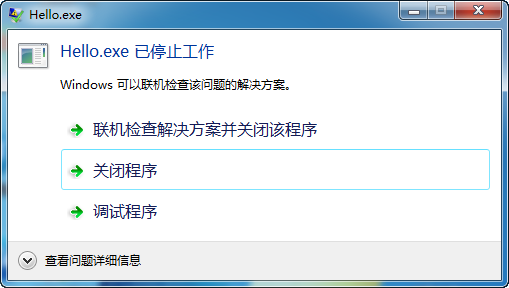* 按F5启动调试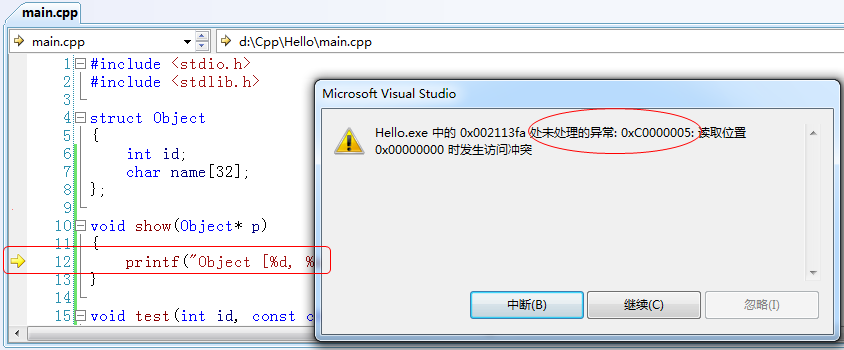- 提示的错误为“未处理的异常 0XC000005，读取位置0x00000000时发生访问冲突”。以后凡是看到这种提示，表示错误的原因是“空指针”。
- 在代码编辑器，黄色箭头已经指向了错误的行。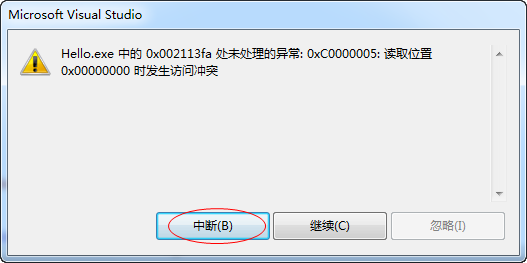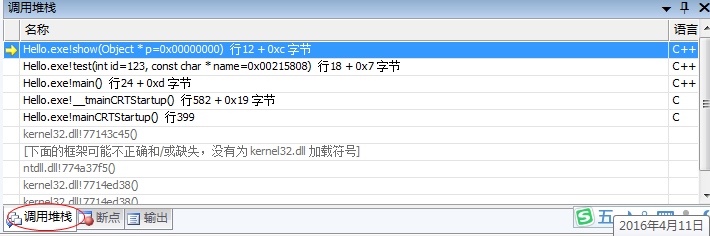二、“调用堆栈”窗口的使用方法

“调用堆栈”窗口里可以观察到：
- 函数的调用层次 ：main() -> test(id, name) ->show(p)
- 每一次函数里的局部变量(含参变量)的值
- 全部变量的值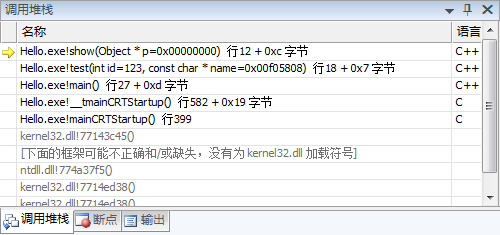（*）从上到下，依次是函数的调用层次
（*）每一行由以下信息组成
Hello.exe!show(Object* p=0x00000000)行12+0xc字节

（3）双击某个函数，可以看到这个函数内的局部变量的值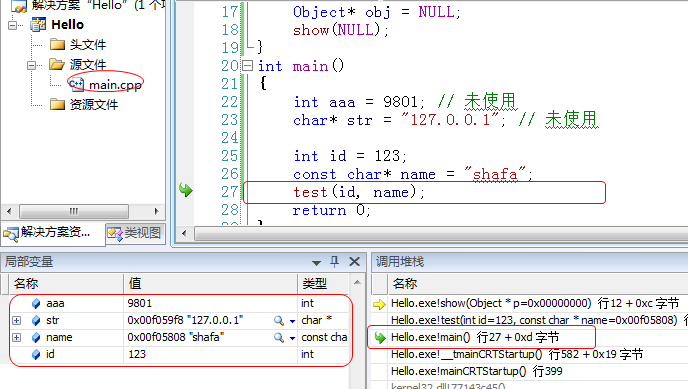三、程序崩溃的原因分类

1. 函数栈溢出
一个变量未初化、未赋值，就读取它的值。
（ 这属于逻辑问题，往往是粗心大意的导致的 ）
2. 函数栈溢出
（1）定义了一个体积太大的局部变量
（2）函数嵌套调用，层次过深（如无穷递归）
3. 数组越界访问
访问数组元素时，下标越界
4. 指针的目标对象不可用
（1）空指针
（2）野指针
• 指针未赋值
• free/delete释放了的对象
• 不恰当的指针强制转换

3.1 读取未赋值的变量

//////////////// 示例 //////////////////
#include <stdio.h>
#include <stdlib.h>
// 求两数的积
int multiply(int m, int n)
{
return m * n;
}
int main()
{
int a, b;
int m = multiply(a, b);//<--这里有错
printf("result: %d \n", m);
return 0;
}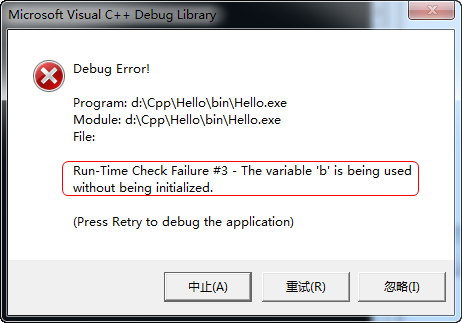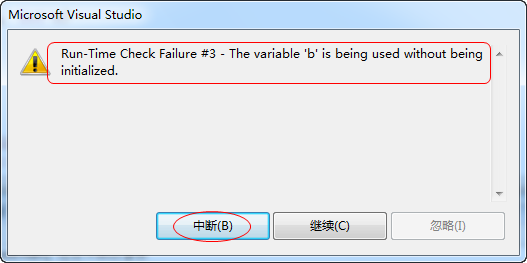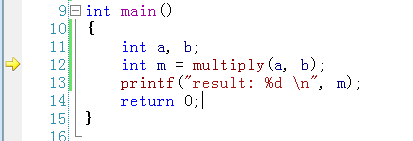3.2 函数栈溢出

（1）定义了一个体积太大的局部变量
（2）函数嵌套调用，层次过深（如无穷递归）

//////////////////// 示例 //////////////////////
#include <stdio.h>
#include <stdlib.h>

// 局部变量的体积太大
void test()
{
int buf[1024*1024*16];  // 这个变量体积太大
printf("DO NOT define a very large buffer on the stack!");
for(int i = 0; i<sizeof(buf)/sizeof(int); i++)
{
buf[i] = i;
}
}
int main()
{
test();
return 0;
}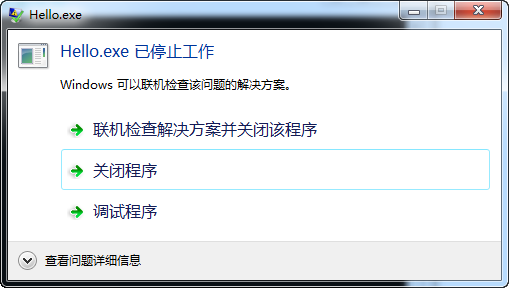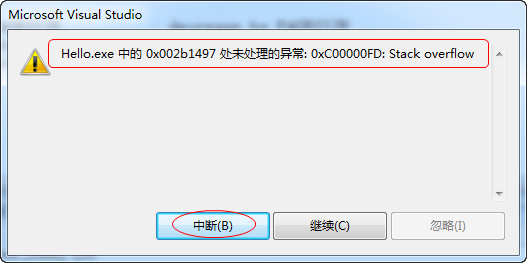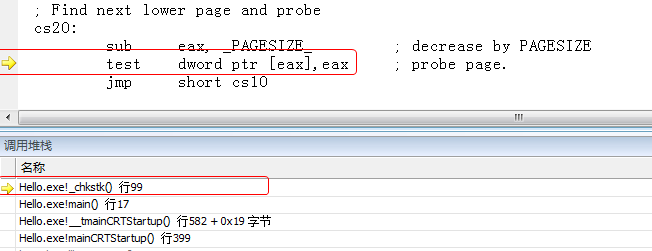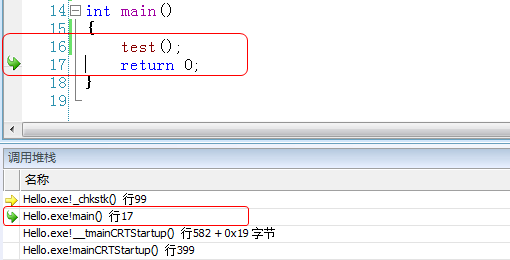///////////////// 示例 /////////////////
#include <stdio.h>
#include <stdlib.h>
void a();
void b();

void a()
{
printf("Calling a() ...\n");
b();
}
void b()
{
printf("Calling b() ...\n");
a();
}
int main()
{
a();
return 0;
}


3.3 数组越界访问

#include <stdio.h>
#include <stdlib.h>
void test(char* p)
{
for(int i=0; i<5; i++)//<--这里有错
{
p[i] *= 10;
printf("%d \n", p[i]);
}
}
int main()
{
char buf = {1,2,3,4};
test(buf);
return 0;
}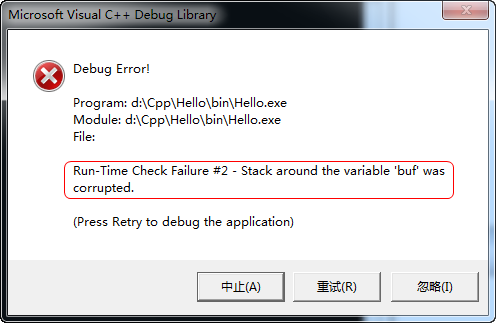3.4指针的目标对象不可用

（1）空指针
（2）野指针
- 指针未赋值
- free/delete释放了的对象
- 不恰当的指针强制转换

3.4.1 空指针

#include <stdio.h>
#include <stdlib.h>
struct Object
{
int id;
char name;
};
void show(Object* p)
{
printf("Object [%d, %s] \n", p->id, p->name);
}
void test(int id, const char* name)
{
Object* obj = NULL;
show(NULL);//<--这里有错
}
int main()
{
int id = 123;
const char* name = "shafa";
test(id, name);
return 0;
}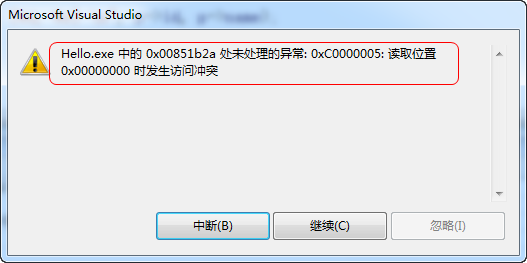3.4.2 野指针: 指针未赋值

////////////////// 示例代码 ///////////////
#include <stdio.h>
#include <stdlib.h>
struct Object
{
int id;
char name;
};
void show(Object* p)
{
printf("Object [%d, %s] \n", p->id, p->name);
}

int main()
{
Object* p;
show(p);//<--这里有错
return 0;
}


The variable is being used without being initialized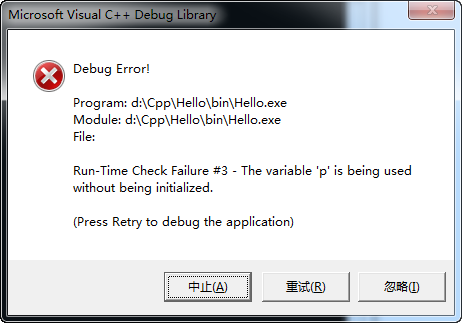3.4.3 野指针: free/delete释放了的对象

#include <stdio.h>
#include <stdlib.h>
#include <string.h>
struct Object
{
int id;
char name;
};

void show(Object* p)
{
printf("Object [%d, %s] \n", p->id, p->name);
}
int main()
{
Object* p = (Object*)malloc(sizeof(Object));
p->id = 123;
strcpy(p->name, "邵发");

free(p); // p指向的内存被释放

p->id = 12; //<--这里有错,不可再对其访问
show(p);
return 0;
}


[参考习题 #145]

3.4.4 野指针：不恰当的指针强制转换

#include <stdio.h>
int main()
{
int a = 10;
double* p = (double*) &a;
*p = 123.345; // 程序崩溃
return 0;
}